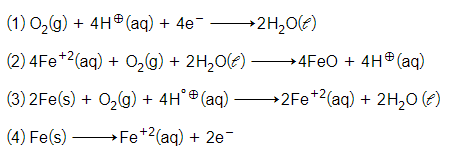What will be the value of $∆\mathrm{G}°$ for the rection

if 

(1) -65.62 KJ

(2) -75.27 KJ

(3) -150.54 KJ

(4) +150.54 KJ

Concept Questions :-

Electrode Potential / Introduction
High Yielding Test Series + Question Bank - NEET 2020

Difficulty Level:

At 298 K the emf of following cell is :-

Pt|H2(1atm)|${\mathrm{H}}^{\oplus }$(0.02M)||${\mathrm{H}}^{\oplus }$(0.01M)|H2(1atm)|Pt

1. - 0.017 V

2. 0.0295 V

3. 0.1 V

4. 0.059 V

Concept Questions :-

Nernst Equation
High Yielding Test Series + Question Bank - NEET 2020

Difficulty Level:

At 25$°$C temperature Zn electrode is placed in 0.1M solution of zinc salt then what will be the oxidation potential ? If it is a assumed that salt dissociates 25% at this dilution ()

(1) -0.713 V

(2) + 0.81 V

(3) + 0.778 V

(4) - 0.84 V

Concept Questions :-

Nernst Equation
High Yielding Test Series + Question Bank - NEET 2020

Difficulty Level:

The reduction potential of Hydrogen electrode is -118mV then the concentration of ${\mathrm{H}}^{\oplus }$ion in solution will be :-

(1) 10-4M

(2) 2M

(3) 0.01M

(4) 10-3M

Concept Questions :-

Nernst Equation
High Yielding Test Series + Question Bank - NEET 2020

Difficulty Level:

What will be the maximum work which can be obtained from a Daniel cell -

1. 106.15 KJ

2. -212.3 KJ

3. 424.6 KJ

4. +212.3 KJ

Concept Questions :-

Electrode Potential / Introduction
High Yielding Test Series + Question Bank - NEET 2020

Difficulty Level:

CuSO4 solution is treated separately with KCl and KI. In which case, Cu+2 will be reduced to ${\mathrm{Cu}}^{\oplus }$ ?

(1) with KCl

(2) with KI

(3) with KCl and KI both

(4) None of these

Concept Questions :-

Electrode Potential / Introduction
High Yielding Test Series + Question Bank - NEET 2020

Difficulty Level:

How many coulombs are required to oxidise 1 mol H2O2 to O2 ?

(1) 9.65 X 104 C

(2) 9.3 X 104 C

(3) 1.93 X 105 C

(4) 19.3 X 102 C

High Yielding Test Series + Question Bank - NEET 2020

Difficulty Level:

The quantity of electricity required to reduce 12.3g of nitrobenzene to aniline with 50% current efficienct is:-

1. 1 F

2. 0.6 F

3. 0.5 F

4. 1.2 F

Concept Questions :-

High Yielding Test Series + Question Bank - NEET 2020

Difficulty Level:

Electrolysis of hot aqueous solution of NaCl gives NaClO4 as-

How many faraday are required to obtain 1000 g of sodium perchlorate ?

(1) 66 F

(2) 122.5 F

(3) 15.13 F

(4) 1.86 F

Concept Questions :-

High Yielding Test Series + Question Bank - NEET 2020

Difficulty Level:

The cell reaction of micro electrochemical cell fromed during rusting of iron is:-Concept Questions :-

Corrosion